ANGSD: Analysis of next generation Sequencing Data

Latest tar.gz version is (0.938/0.939 on github), see Change_log for changes, and download it here.

# ErrorPerfect

## Method 2

The method was use in Orlando2013 and is described in details in supplementary 4.4

The estimated rates can roughly be intrepreted as relative error rates. That is excess of errors in your sample compare to the error in a high quality indvidual. The idea is the your sample and the high quality individual should have the same expected number of derived alleles and the extra derived alleles in you sample are due to the excess error. We use only positions were there are coverage for both the chimp, the sample and the high quality genome. The overall error rate is obtained from$O_D = E_D(1-\epsilon) + E_A\epsilon$

were

•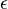$\epsilon$ is the error rate
•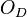$O_D$ is the observed number of derived alleles in the sample
•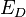$E_D$ is the expected number of derived alleles which is obtained from the observed derived alleles from the high quality genome
•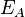$E_A$ is the expected number of ancestral alleles which is obtained from the high quality genome

The type specific error rates are obtained from maximizing the likehood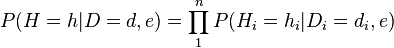$P(H=h|D=d,e) = \prod_1^n P(H_i=h_i|D_i=d_i,e)$ where n is the number of sampled bases,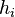$h_i$ is the observed base of the sample,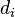$d_i$ is the obsered base of the outgroup and e is an error rate matrix with entrance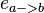$e_{a->b}$ being the rate of error from base a to different base b and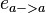$e_{a->a}$ being equal to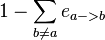$1-\sum_{b\ne a} e_{a->b}$

We calculate the above by summing over the true unobserved base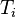$T_{i}$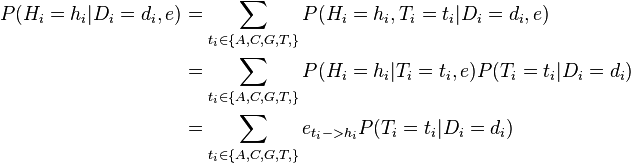\begin{align} P(H_i=h_i|D_i=d_i,e) &= \sum_{t_i\in\{A,C,G,T,\}} P(H_i=h_i,T_i=t_i|D_i=d_i,e) \\ &= \sum_{t_i\in\{A,C,G,T,\}} P(H_i=h_i|T_i=t_i,e)P(T_i=t_i|D_i=d_i) \\ &= \sum_{t_i\in\{A,C,G,T,\}} e_{t_i->h_i}P(T_i=t_i|D_i=d_i) \end{align}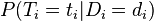$P(T_i=t_i|D_i=d_i)$ is set equal to the observed fraction of sites in the high quality genome where the outgroup has base$d_i$ and the high qualtiy genome has base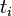$t_i$